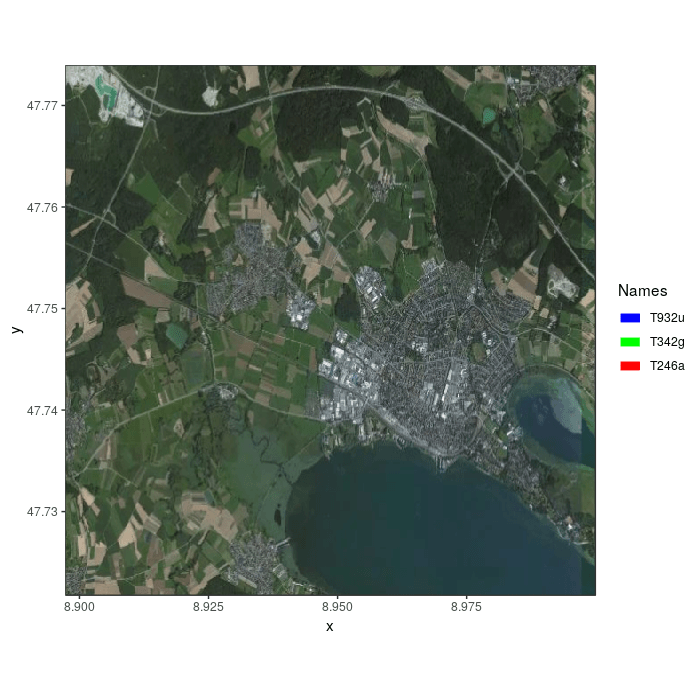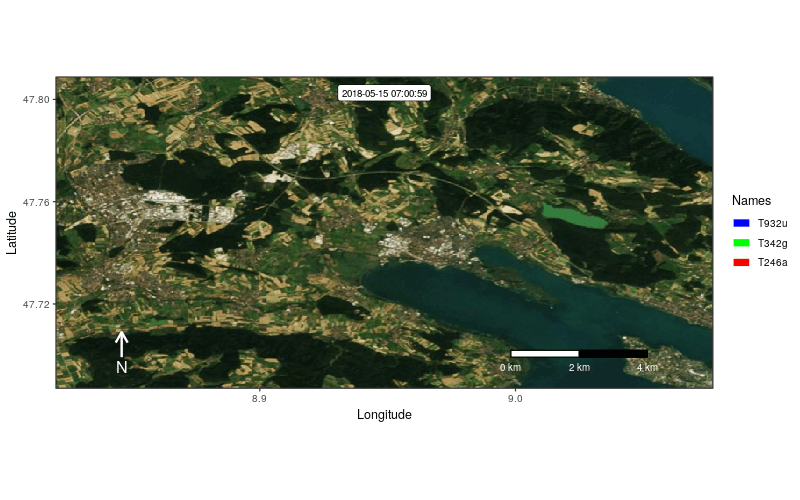Thanks to the slippymath package used by frames_spatial, you can use mapbox base maps (e.g. satellite):

library(moveVis)
library(move)

data("move_data")

# align movement to unique times and regular resolution
m <- align_move(move_data, res = 4, unit = "mins")

## assign some path colours by individual
m.list <- split(m) # split m into list by individual
m.list <- mapply(x = m.list, y = c("red", "green", "blue"), function(x, y){
x\$colour <- y
return(x)
}) # add colour per individual
m <- moveStack(m.list) # putting it back together into a moveStack

# create frames with mapbox satellite basemap
frames <- frames_spatial(m, map_service = "mapbox", map_type = "satellite",
map_token = "YOUR_MAPBOX_TOKEN")
# register at http://www.mapbox.com to get a free mapbox token
# that allows you to do 50.000 map requests per month free of charge

# animate the first 100 frames as example
animate_frames(frames[1:100], out_file = "example_3a.gif")In case, you do not want to force the calculation of an equidistant (squared) extent with x and y axis representing equal surface distances, you can turn it off by setting equidistant = FALSE.

# to not calculate a squared (enlarged) extent:
frames <- frames_spatial(m, map_service = "mapbox", map_type = "satellite",
map_token = "YOUR_MAPBOX_TOKEN", equidistant = FALSE)

You can also use a custom extent (and use the pipe to customize frames):

ext <- extent(8.820289, 9.076893, 47.68715, 47.80863)

# set the ext argument
frames <- frames_spatial(m, map_service = "mapbox", map_type = "satellite",
map_token = "YOUR_MAPBOX_TOKEN", ext = ext, equidistant = FALSE) %>%
add_labels(x = "Longitude", y = "Latitude") %>%
add_northarrow(colour = "white", height = 0.08, position = "bottomleft") %>%
add_scalebar(colour = "white", height = 0.022, position = "bottomright", label_margin = 1.4) %>%
height = 500, width = 800, res = 82)For further details, see ?frames_spatial.